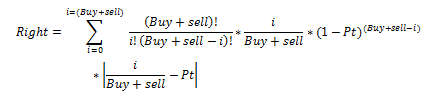## Iterative sum

Hi everyone,

I'm facing an  issue when doing an itrative sum using factorials. This is the first time I use both an iterative sum and the FACT function so I guess there are a  lot of things wrong in my code.

Want I want is the formula I hereby attached. The output should always be between 0 and 1 since Pt is always around 0,5. Nevertheless ith my formula I obtain totlly different numbers that can be super high.

Here is my code:

dATA LSV.right;

set lsv.left;

right=0;

do i=0 to n;

calc1=fact(n) / fact(i) / fact(n-i);

calc2= calc1 * i/n ;

calc3=calc2* (1-Pt)**(n-i) ;

calc4= calc3* abs ((i/n) -Pt);

right=calc4 + right;

abs=abs((i/n)-Pt);

Prod=calc1*calc2*calc3*calc4;

end;

run;1 ACCEPTED SOLUTION

Accepted Solutions

## Re: Iterative sum

It's possible that you are running into overflow issues, in which case you'll need to be more careful about the order of operations.

What sort of values are you expecting, and what are you getting instead?  (We don't have your data.)

What is your formula supposed to calculate?  Are you maybe missing a Pt**i factor?

6 REPLIES 6

## Re: Iterative sum

Looks like you are multiplying everything twice.  You can just skip the last two lines (abs and Prod) in the body of the loop.

Also, you can use the COMB function to compute calc1 directly, without using FACT.

## Re: Iterative sum

Thanks I've corrected my formula but it's still giving me the same numbers that are way  too high.

dATA LSV.right;

set lsv.left;

right=0;

do i=0 to n;

right= comb(n,i)* (i/n) * ((1-Pt)**(n-i)) * abs ((i/n) -Pt) +right;

end;

run;

## Re: Iterative sum

It's possible that you are running into overflow issues, in which case you'll need to be more careful about the order of operations.

What sort of values are you expecting, and what are you getting instead?  (We don't have your data.)

What is your formula supposed to calculate?  Are you maybe missing a Pt**i factor?

## Re: Iterative sum

A colleague has suggested using a log transformation to reduce the scale and help avoid overflow.  He sent this code:

data  left;
input n pt;
cards;
1000 0.9995
1000 0.95
1000 0.5
1000 0.005
run;
data right;
set left;
sum = 0;

/****************************************************/
/* when i = 0 term = 0                              */
/* the last term is always bounded by max(1,abs(pt) */
/* with pt small log1px(-pt) should be used         */
/* consider using lbeta instead of lfacts           */
/****************************************************/
log1mpt = log(1-pt);
do i=0 to n;
if (i = 0) then       logterm = -10000000;
else  do;
logterm = lfact(n) - lfact(i) - lfact(n-i)  + log(i/n) + (n-i) * log1mpt;
end;
sum= sum + exp(logterm) * abs(i/n-pt);
end;
put sum=;
run;

## Re: Iterative sum

Thanks a lot.  i just fund out my problem. I was a misintepretation of the formula. As you said earlier I  had forgotten a  Pt**i factor.

Thank you very much for your precious help.